# MISSION BANKING 2023 Reasoning Ability Quiz – 22

## MISSION BANKING 2023 Reasoning Ability Quiz

Aspirants have a strong possibility of scoring well in the Reasoning Ability section if they practice quality questions on a regular basis. This section takes the least amount of time if the practice is done every day in a dedicated manner. In this article, we have come up with the MISSION BANKING 2023 Reasoning Ability Quiz to help you prepare better. Candidates will be provided with a detailed solution for each question in this MISSION BANKING 2023 Reasoning Ability Quiz. This MISSION BANKING 2023 Reasoning Ability Quiz includes a variety of questions ranging in difficulty from easy to tough. This MISSION BANKING 2023 Reasoning Ability Quiz is totally FREE. This MISSION BANKING 2023 Reasoning Ability Quiz has important Reasoning Ability Questions and Answers that will help you improve your exam score. Aspirants must practice this MISSION BANKING 2023 Reasoning Ability Quiz in order to be able to answer questions quickly and efficiently in upcoming exams.

Directions (1-5): Each of the questions below, consist a question and three statements numbered I, II and III. You have to decide whether the data provided in the statements are sufficient to answer the question. Read the three statements and Give answer

1. Among eight friends A, B, C, D, P, Q, R and S, who is sitting between B and R? (it is given that all the friends are sitting around circular table facing out­side the centre of the table).

Statements: I. S is seated third to the left of D, who is not near to A.

II. P is seated immediate right of D and third to the right of R, who is near to C.

III. Q is seated third to the left of A, who is second to the right of B, who is not near to C.

(a) If the data in statement I and II together are sufficient to answer the question, while the data in statement III are not required to answer the question.

(b) If the data in statement I and III together are sufficient to answer the question, while the data in statement II are not required to answer the question.

(c) If the data in statement II and III are sufficient to answer the question, while the data in statement I are not required to answer the question.

(d) If the data in all three statements I, II and III together are necessary to answer the question.

(e) If the data in all the statements, I, II and III even together are not sufficient to answer the question.

Ans. c

Exp.  By using II and III statement we can arrange all the persons in a circular arrangement, so we can now know, who sits between ‘B’ and ‘R’.

1. What is the code for ‘RUN’ in a certain code language?

Statements :  I. In a certain code language ‘run your business’ is coded as ‘lap duh pud’ and ‘mind your language’ is coded as ‘hpu dap lap’.

II. In a certain code language ‘run fast and win’ is coded as ‘phl pud lph hap’ and ‘go and move ahead’ is coded as ‘hap pul pah lph’

III. In a certain code language ‘move your legs’ is coded as ‘pah lap plh’ and ‘legs go on’ is coded as ‘pal plh pul’

(a) If the data in statement I and II together are sufficient to answer the question, while the data in statement III are not required to answer the question.

(b) If the data in statement I and III together are sufficient to answer the question, while the data in statement II are not required to answer the question.

(c) If the data in statement II and III are sufficient to answer the question, while the data in statement I are not required to answer the question.

(d) If the data in all three statements I, II and III together are necessary to answer the question.

(e) If the data in all the statements, I, II and III even together are not sufficient to answer the question.

Ans. a

Exp.  By using I and II statement, we can find the code of run. RUN-‘pud’

1. Point X is in which direction with respect to J ?

Statements :        I. Point S is to the west of point T, which is in south of point J. Point X is to the north of point S.

II. Point J is to the east of point V, which is the south of point T. Point X is to the south of point W.

III. Point T is to the west of point Y, which is to the north of point W.

(a) If the data in statement I and II together are sufficient to answer the question, while the data in statement III are not required to answer the question.

(b) If the data in statement I and III together are sufficient to answer the question, while the data in statement II are not required to answer the question.

(c) If the data in statement II and III are sufficient to answer the question, while the data in statement I are not required to answer the question.

(d) If the data in all three statements I, II and III together are necessary to answer the question.

(e) If the data in all the statements, I, II and III even together are not sufficient to answer the question.

Ans. e

1. Eight students A, B, C, D, E, F, G and H are sitting around a circle facing to the centre of the table. How many students are sitting between C and F ?

Statements :        I. A is seated second to the right of H, who is seated third to the right of D. G is seated immediate left of F.

II. C is seated third to the right of A and is not near to E. F is seated second to the right B.

III. D is seated second to the left of E and is third to right of G. C is an immediate neighbor of H.

(a) If the data in statement I and II together are sufficient to answer the question, while the data in statement III are not required to answer the question.

(b) If the data in statement I and III together are sufficient to answer the question, while the data in statement II are not required to answer the question.

(c) If the data in statement II and III are sufficient to answer the question, while the data in statement I are not required to answer the question.

(d) If the data in all three statements I, II and III together are necessary to answer the question.

(e) If the data in all the statements, I, II and III even together are not sufficient to answer the question.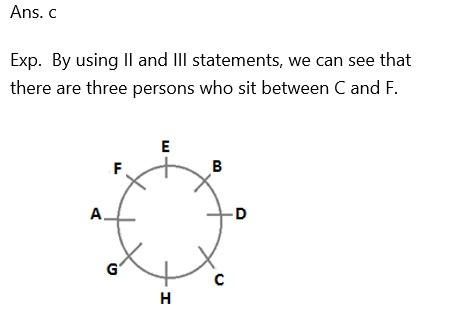1. Who earns highest salary among six members in a family? (six members A, B, C, D, E and F are earning different salaries)
Statements : I. A earns more than only C and D. F earns more than B.

II. B earns less than E while D earns more than A and F.

III. C earns more than E but less than D. F earns more than two persons.

(a) If the data in statement I and II together are sufficient to answer the question, while the data in statement III are not required to answer the question.

(b) If the data in statement I and III together are sufficient to answer the question, while the data in statement II are not required to answer the question.

(c) If the data in statement II and III are sufficient to answer the question, while the data in statement I are not required to answer the question.

(d) If the data in all three statements I, II and III together are necessary to answer the question.

(e) If the data in all the statements, I, II and III even together are not sufficient to answer the question.

Ans. c

Exp.  By using II and III statements, we can see that, D earns the highest salary.

D>C>E>B

D>A and F

Directions (6-8): In each of the questions given below some statements are followed by some conclusions. You have to take the given statements to be true even if they seem to be at variance from commonly known facts. Read all the conclusions and then decide which of the given conclusions logically follows from the given statements disregarding commonly known facts.

1. Statements: Some myntra is flipkart

Some flipkart is jaboong

No jaboong is snapdeal

All snapdeal is shopclues

Conclusions:       I. Some flipkart is not snapdeal

II. no shopclues is jaboong

III. All snapdeal can be shopclues

(a)Only I and II follow

(b)Only II and III follow

(c)Only I and III follow

(d)All follow

(e)None of the above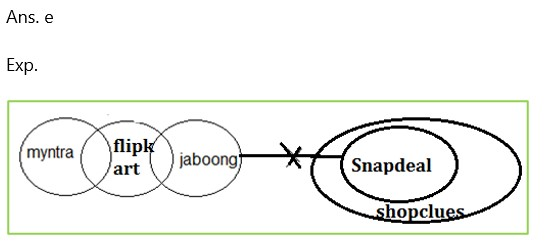1. Statements: Some dog is cat.

All cat is lion

All lion is tiger

No tiger is ant

Some honeybee is ant

Conclusions:                        I. No cat is ant

II. Only tiger is cat

III. Some ant is not lion.

IV. No cat is honeybee

(a) Only I and III follow

(b) Only I and II follow

(c) Only III and IV follow

(d)None follows

(e)All follows except IV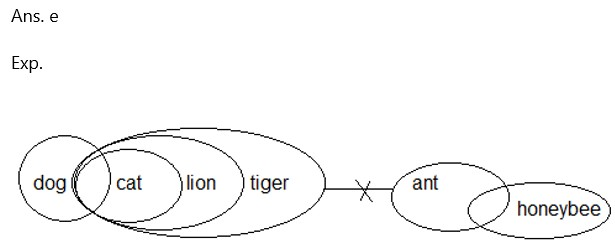1. Statements: No pluto is jupiter

No Jupiter is saturn

Some mercury is Jupiter

All mercury is venus

Conclusions:                        I. Some Saturn can never be jupiter

II. No venus is saturn

III. No pluto is saturn

IV. Some Jupiter is venus.

(a)Only I and IV follow

(b)Only I and II follow

(c)Only III and IV follow

(d) None of these.

(e)Only I follow.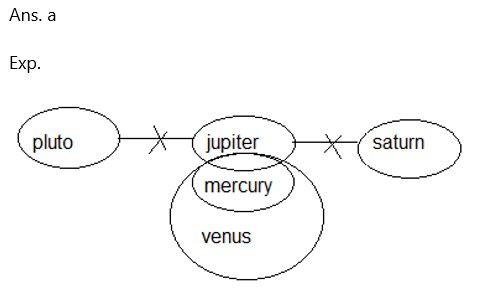Directions (9-10): Answer these questions based on the following information.

In a certain code:

“document fly high bus” is coded as – “ lt lo gh us”

“quota bus conductor classic” is coded as – “us to dc se”

“quota fly bus talent ” is coded as – “lo us to ln”

“march high conductor classic ” is coded as – “mn dc se  gh”

1. What will be the code for “conductor”?

(a) mn

(b) dc

(c) se

(d) can’t be determined

(e) gh

Ans. d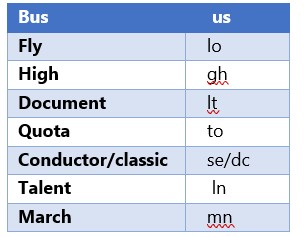1. What may be the code for “bus document talent juice”?

(a) us lt ln mn

(b) us lt ln jd

(c) ln lo to gh

(d) mn us gh se

(e) None of these

Ans. b### 2023 Preparation Kit PDF

#### Most important PDF’s for Bank, SSC, Railway and Other Government Exam : Download PDF Now

AATMA-NIRBHAR Series- Static GK/Awareness Practice Ebook PDF Get PDF here
The Banking Awareness 500 MCQs E-book| Bilingual (Hindi + English) Get PDF here
AATMA-NIRBHAR Series- Banking Awareness Practice Ebook PDF Get PDF here
Computer Awareness Capsule 2.O Get PDF here
AATMA-NIRBHAR Series Quantitative Aptitude Topic-Wise PDF 2020 Get PDF here
Memory Based Puzzle E-book | 2016-19 Exams Covered Get PDF here
Caselet Data Interpretation 200 Questions Get PDF here
Puzzle & Seating Arrangement E-Book for BANK PO MAINS (Vol-1) Get PDF here
ARITHMETIC DATA INTERPRETATION 2.O E-book Get PDF here

3